/scikit-learn

# sklearn.metrics.confusion_matrix

`sklearn.metrics.confusion_matrix(y_true, y_pred, labels=None, sample_weight=None)` [source]

Compute confusion matrix to evaluate the accuracy of a classification

By definition a confusion matrix \(C\) is such that \(C_{i, j}\) is equal to the number of observations known to be in group \(i\) but predicted to be in group \(j\).

Thus in binary classification, the count of true negatives is \(C_{0,0}\), false negatives is \(C_{1,0}\), true positives is \(C_{1,1}\) and false positives is \(C_{0,1}\).

Read more in the User Guide.

Parameters: `y_true : array, shape = [n_samples]` Ground truth (correct) target values. `y_pred : array, shape = [n_samples]` Estimated targets as returned by a classifier. `labels : array, shape = [n_classes], optional` List of labels to index the matrix. This may be used to reorder or select a subset of labels. If none is given, those that appear at least once in `y_true` or `y_pred` are used in sorted order. `sample_weight : array-like of shape = [n_samples], optional` Sample weights. `C : array, shape = [n_classes, n_classes]` Confusion matrix

#### References

  Wikipedia entry for the Confusion matrix (Wikipedia and other references may use a different convention for axes)

#### Examples

```>>> from sklearn.metrics import confusion_matrix
>>> y_true = [2, 0, 2, 2, 0, 1]
>>> y_pred = [0, 0, 2, 2, 0, 2]
>>> confusion_matrix(y_true, y_pred)
array([[2, 0, 0],
[0, 0, 1],
[1, 0, 2]])
```
```>>> y_true = ["cat", "ant", "cat", "cat", "ant", "bird"]
>>> y_pred = ["ant", "ant", "cat", "cat", "ant", "cat"]
>>> confusion_matrix(y_true, y_pred, labels=["ant", "bird", "cat"])
array([[2, 0, 0],
[0, 0, 1],
[1, 0, 2]])
```

In the binary case, we can extract true positives, etc as follows:

```>>> tn, fp, fn, tp = confusion_matrix([0, 1, 0, 1], [1, 1, 1, 0]).ravel()
>>> (tn, fp, fn, tp)
(0, 2, 1, 1)
```

## Examples using `sklearn.metrics.confusion_matrix`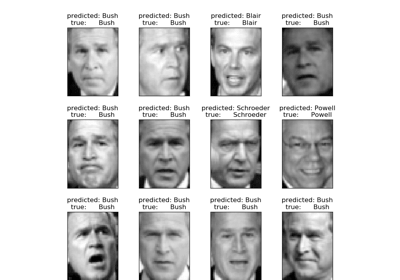Faces recognition example using eigenfaces and SVMs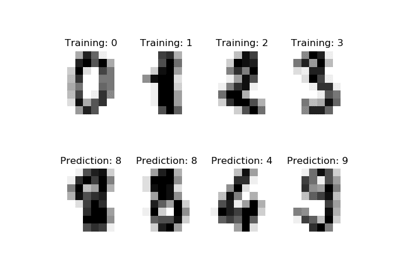Recognizing hand-written digits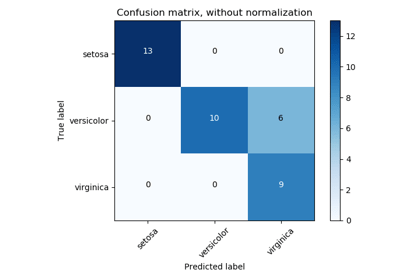Confusion matrix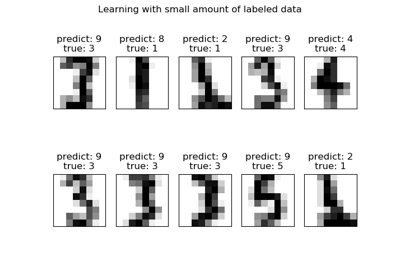Label Propagation digits: Demonstrating performance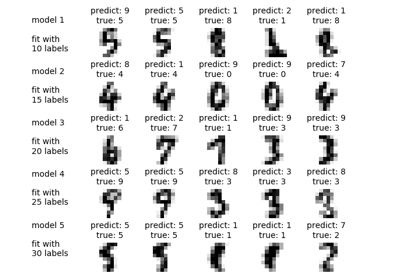Label Propagation digits active learning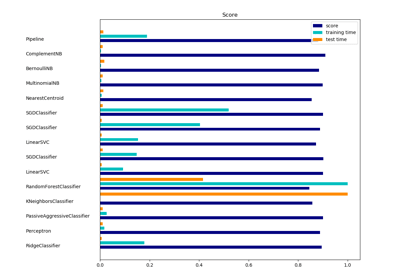Classification of text documents using sparse features